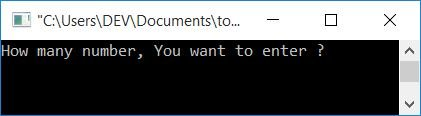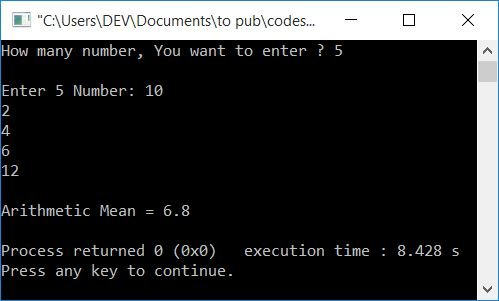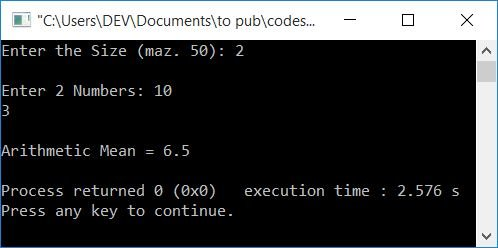# C++ Program to Calculate Arithmetic Mean

In this article, you will learn and get code on finding the arithmetic of all the numbers entered by the user in C++. The user is also allowed to define the size. For example, if the user enters 5 as the size, then the program further asks for any 5 numbers to find the arithmetic mean. There are two programs available here:

### How to find the arithmetic mean

If there are n sets of numbers, we have a formula for calculating the arithmetic mean:

`am = (n1+n2+n3+...+nn)/n`

Here am indicates the arithmetic mean, whereas n1, n2, n3, and nn indicate the first, second, third, and last number, respectively.

For example, if we have five sets of numbers, say 10, 20, 30, 40, and 50, we can calculate their arithmetic mean as follows:

```am = (10+20+30+40+50)/5
= 150/5
= 30```

Now let's move on to the program.

## In C++, find the Arithmetic Mean

In order to calculate (or find) the arithmetic mean (of numbers) in C++ programming, you must first ask the user to enter the size (how many sets of numbers) and then ask the user to enter all numbers of that size in order to find and print the arithmetic mean.

To calculate the arithmetic mean of numbers, first perform the addition of all the numbers, then make a variable  responsible for the arithmetic mean and place addition or size in a variable saying "armean" (arithmetic mean), then display the result on the output screen as shown here in the following program.

```#include<iostream>
using namespace std;
int main()
{
int n, i;
float arr, sum=0, armean;
cout<<"How many number, You want to enter ? ";
cin>>n;
cout<<"\nEnter "<<n<<" Number: ";
for(i=0; i<n; i++)
{
cin>>arr[i];
sum = sum+arr[i];
}
armean = sum/n;
cout<<"\nArithmetic Mean = "<<armean;
cout<<endl;
return 0;
}```

This program was built and runs under the Code::Blocks IDE. Here is its sample run:Now enter the size, which is how many numbers you want to enter to find the arithmetic mean. Let's suppose, user enters the size as 5 and the 5 numbers as 10, 2, 4, 6, and 12. Press the ENTER key to see the following output:When we received the numbers, we added them one by one to the total. For instance, if the user enters 5 as the size and the numbers 10, 2, 4, 6, and 12, the dry run (within the for loop) will look like this:

• Initially, 0 gets initialized to i. So i=0
• Checks the condition to see if i (0) is less than n (5).
• The condition evaluates to be true, therefore program flow goes inside the loop and receives a number from the user and stores it in arr[i] (that is, arr).
• And the statement sum = sum+arr[i] or sum = 0+arr or sum = 0+10 (assuming the first number is 10). Therefore, sum=10
• The program flow now moves to the for loop's updating section and increments the value of i. Now i=1
• Checks the condition because condition was evaluated as true again, so program flow returns to the loop and evaluates two statements.
• that is, receives a number, say 2, and stores it in arr.
• Similarly, sum+arr or 10+2 is initialized to sum. Now sum=12
• Continue until the value of i equals 5 or the condition i<n evaluates to false.
• After exiting from the loop, we'll have a variable sum that holds the sum of all the 5 numbers.
• Now apply the final formula of arithmetic mean, which is sum/size, which is sum/5. Put the sum value. The variable armean holds its value (sum/size). Therefore, just print the value of armean as output, that will be the arithmetic mean.

### In C++, find the arithmetic mean using the function

This function does the same job as the previous program. But it is created using functions. That is, a function like arithmeticMean() takes two arguments: the first one is the array, and the second is its size. and returns the arithmetic mean of all numbers stored in the array.

```#include<iostream>
using namespace std;
float arithmeticMean(float [], int);
int main()
{
int n, i;
float arr, armean;
cout<<"Enter the Size (maz. 50): ";
cin>>n;
cout<<"\nEnter "<<n<<" Numbers: ";
for(i=0; i<n; i++)
cin>>arr[i];
armean = arithmeticMean(arr, n);
cout<<"\nArithmetic Mean = "<<armean;
cout<<endl;
return 0;
}
float arithmeticMean(float arr[], int n)
{
int i;
float sum=0, am;
for(i=0; i<n; i++)
sum = sum+arr[i];
am = sum/n;
return am;
}```

Here is its sample run with user input of 2 as size and 10, 3, as two numbers:#### The same program in different languages

C++ Quiz

« Previous Program Next Program »